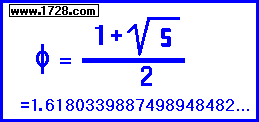FibonacciNumber Trick
 Here is a number trick that is not too well known and so you can use it to impress your friends. In fact this doesn't require a computer and can even be done with paper and pencil. It involves a series known as the Fibonacci number sequence, named after the Italian mathematician Leonardo Fibonacci (1170-1250).
 A Fibonacci number sequence is formed by starting with any two numbers, adding those to get a third number, adding the second and third to produce a fourth number and so on. This is much easier to see with a short example:       2       3       5       8     13     21     34     55     89   144
As can be seen, the sequence is formed by adding the previous two numbers.
2 plus 3 = 5, 3 plus 5 = 8, 5 plus 8 = 13, 8 plus 13 = 21 and so on.
Using the calculator below, if you input 2 and 3 into the first two boxes, when you click "Calculate", you will see all 10 boxes filled in with the same numbers in the list above.

Now, for the "trick" with the Fibonacci number sequence.
You could then enter the numbers in this computer page but it is much more impressive if this trick is done on paper.
Adding the two numbers, create a Fibonacci sequence that is exactly ten steps long.
When you reach the tenth number, tell your friend you can total all ten numbers in your head!

And what's the secret?

Whenever you have a Fibonacci sequence of 10 numbers, the total will always be the seventh number times 11.

For practice, input two and three in the first two boxes and then click "CALCULATE".
Yes, you could get the total by clicking "Calc Total" or by using the trick.

If you entered two and three for the first two numbers, the seventh number will be 34 and multiplying this by 11 gives a result of 374.
For example, to multiply 34 by 11, think of summing 34 and 34 but shift one number one decimal place.

34
34
374

Obviously, when you ask a friend for two numbers make sure you mention they should be kept relatively small.

Doing this trick on paper is much more impressive than on a computer so why not use this computer page just for practice?

Remember, before you show this to anybody, the best advice is to practice.

* * * * * * * * * * * * * * * *
And, if you are curious, here are the first 100 Fibonacci numbers:

 1 1 2 1 3 2 4 3 5 5 6 8 7 13 8 21 9 34 10 55 11 89 12 144 13 233 14 377 15 610 16 987 17 1,597 18 2,584 19 4,181 20 6,765 21 10,946 22 17,711 23 28,657 24 46,368 25 75,025 26 121,393 27 196,418 28 317,811 29 514,229 30 832,040 31 1,346,269 32 2,178,309 33 3,524,578 34 5,702,887 35 9,227,465 36 14,930,352 37 24,157,817 38 39,088,169 39 63,245,986 40 102,334,155 41 165,580,141 42 267,914,296 43 433,494,437 44 701,408,733 45 1,134,903,170 46 1,836,311,903 47 2,971,215,073 48 4,807,526,976 49 7,778,742,049 50 12,586,269,025 51 20,365,011,074 52 32,951,280,099 53 53,316,291,173 54 86,267,571,272 55 139,583,862,445 56 225,851,433,717 57 365,435,296,162 58 591,286,729,879 59 956,722,026,041 60 1,548,008,755,920 61 2,504,730,781,961 62 4,052,739,537,881 63 6,557,470,319,842 64 10,610,209,857,723 65 17,167,680,177,565 66 27,777,890,035,288 67 44,945,570,212,853 68 72,723,460,248,141 69 117,669,030,460,994 70 190,392,490,709,135 71 308,061,521,170,129 72 498,454,011,879,264 73 806,515,533,049,393 74 1,304,969,544,928,657 75 2,111,485,077,978,050 76 3,416,454,622,906,707 77 5,527,939,700,884,757 78 8,944,394,323,791,464 79 14,472,334,024,676,221 80 23,416,728,348,467,685 81 37,889,062,373,143,906 82 61,305,790,721,611,591 83 99,194,853,094,755,497 84 160,500,643,816,367,088 85 259,695,496,911,122,585 86 420,196,140,727,489,673 87 679,891,637,638,612,258 88 1,100,087,778,366,101,931 89 1,779,979,416,004,714,189 90 2,880,067,194,370,816,120 91 4,660,046,610,375,530,309 92 7,540,113,804,746,346,429 93 12,200,160,415,121,876,738 94 19,740,274,219,868,223,167 95 31,940,434,634,990,099,905 96 51,680,708,854,858,323,072 97 83,621,143,489,848,422,977 98 135,301,852,344,706,746,049 99 218,922,995,834,555,169,026 100 354,224,848,179,261,915,075

An interesting aspect of the Fibonacci Number Sequence is that if you divide one Fibonacci number by the previous Fibonacci number, this produces a quotient called the phi ratio φ which is also known as the golden ratio.

For example, Fibonacci #20 divided by Fibonacci #19 =

6,765 ÷ 4,181 = 1.618033963166...

Fibonacci #50 divided by Fibonacci #49 =

12,586,269,025 ÷ 7,778,742,049 = 1.6180339887499...

As we go further down the Fibonacci sequence, this number approaches a limit of {1 + Square Root (5) } ÷ 2
= 1.6180339887498948482...Another way to express the phi ratio is:
φ = 0.5 + 5.5 • 0.5 = 1.6180339887498948482...

* * * * * * * * * * * * * * * *
The Fibonacci Sequence appears in many places.

Here is a website that explains the arrangement of seeds in a sunflower being based on the Fibonacci Sequence.

* * * * * * * * * * * * * * * *
Leonardo Fibonacci (1170 - 1250) is thought to be the western world's most skillful mathematician of the Middle Ages.
In his 1202 book Libre Abaci he strongly advocated for the use of Arabic Numerals as opposed to Roman Numerals.
He stated that Arabic Numerals were much easier to read and calculations could be done more quickly and accurately with them.